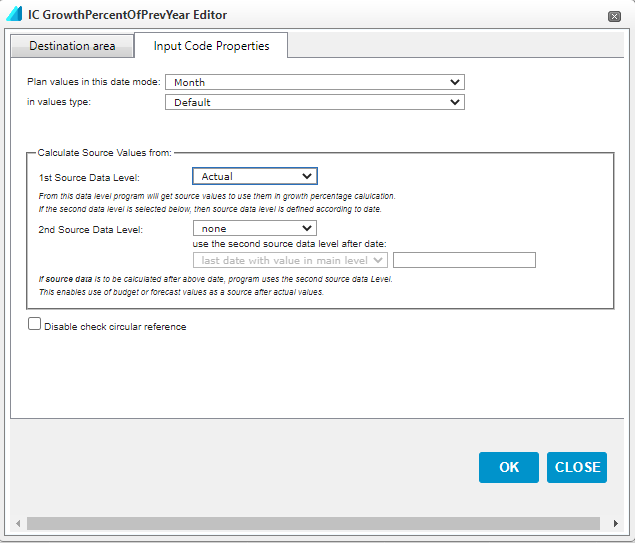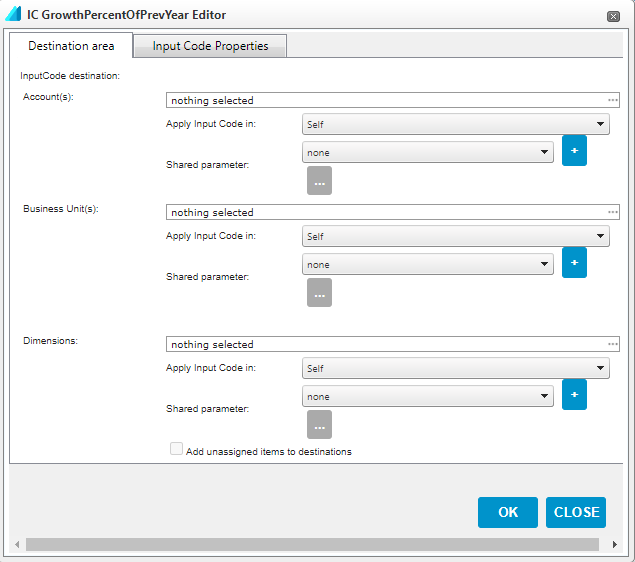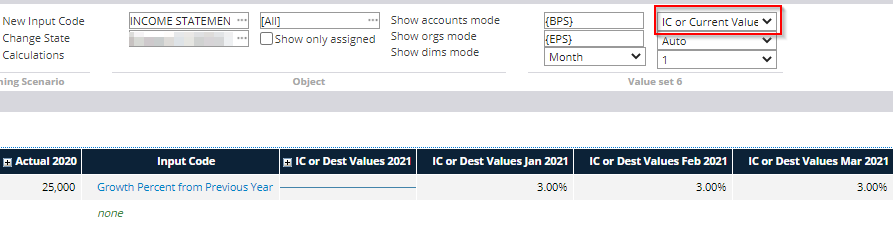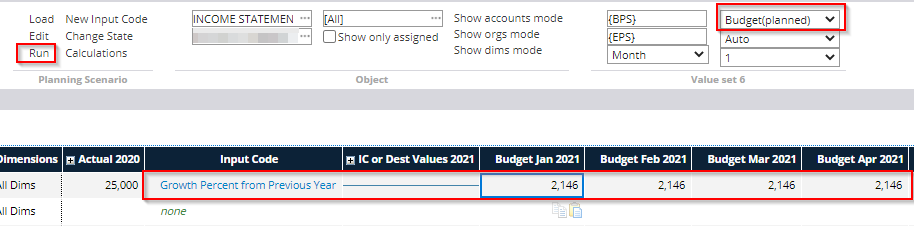The Growth percentage from previous year input code calculates the value according to a certain growth percentage from the corresponding values of the previous financial year.

The Growth percentage from previous period input code always calculates the value according to the growth percentage from the value of the previous period, and not from the corresponding time of the previous financial year. For example, the budgeted value in September will increase by a certain percentage from August (previous period).

Input Code Properties -tabPlan values in this date mode: This setting determines the accuracy level at which the calculation is performed. With monthly accuracy, a separate percentage is determined for each month while, for example, with annual accuracy, only one percentage value is determined for the entire year. This choice also affects the calculation accuracy of the values: monthly accuracy calculates percentages of each month's balance separately, while annual accuracy calculates percentages of the full year's total and divides the result into months based on breakdown rules (e.g., even or proportional distribution).

In values type: Specifies the type of calculated values stored in the system. In most cases, you can use the default setting, in which case the type of values calculated depends on the settings made on the chart of accounts.

1st Source Data Level: Determines from which data level the growth percentage is calculated.

2nd Source Data Level: This option can be used if the data level used to calculate the output values need to be changed after a certain date. If the growth rate is calculated only from one source data level, 'None' should be selected.

Disable check circular reference: Allows the use of circular references in the input code, i.e. references that depend on the results of the same formula.

Destination area -tabAccount(s): Accounts / account groups for which the input code is used.

Business Unit(s): Organizational levels for which the input code is used.

Dimensions: Dimensions or dimension units for which the input code is used. By selecting 'Add unassigned itemsto destinations', the calculation is also performed for values that are not dimensioned.

For all of the above parameters, there are two choices to determine how extensively the calculation is performed:

Apply Input Code in: Specifies whether the input code is used only at the selected level, its cost centers, or parallel levels

Shared parameter: A common parameter that contains user-defined organizational levels or accounts can be selected in this field. This function can be used if you want to add several different input codes to the same set of accounts or organizations, without defining them in each input code separately. A new common parameter is created from the + sign.

Once all the above parameters have been defined, select 'OK' to create the input code.

Entering the input value and viewing the results

After setting the input code properties, a percentage, ie. the input value, must be set as the basis for the calculation. The input value can only be entered in the 'IC or Current Value' mode, which must be selected from the toolbar as shown.

The percentage is entered either in the year column or for individual months, depending on the Plan values in this date mode setting in the properties.

In the example below, the planning accuracy is set to month, in which case the percentage is entered for each month separately. The annual column has a blue line to indicate that a percentage value cannot be entered on an annual basis.Once the percentages have been entered, the actual calculation for the input form is performed by selecting 'Run' from the toolbar.

The values calculated with the input code can be viewed by selecting Budget or Planned as the level mode, depending on whether the input form is for Budget or Forecast.